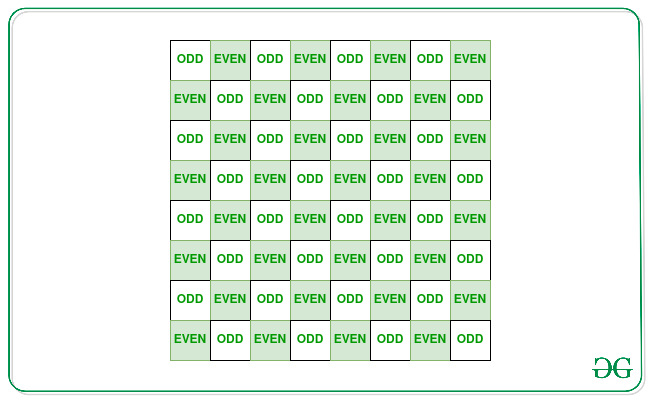# Minimum decrements required to make all pairs of adjacent matrix elements distinct

• Last Updated : 23 Apr, 2021

Given a matrix mat[][] of dimension N * M, the task is to count the minimum number of decrements of distinct array elements required such that no two adjacent matrix elements are equal.

Examples:

Attention reader! Don’t stop learning now. Get hold of all the important mathematical concepts for competitive programming with the Essential Maths for CP Course at a student-friendly price. To complete your preparation from learning a language to DS Algo and many more,  please refer Complete Interview Preparation Course.

Input: mat[][] = { {2, 3, 4}, {2, 5, 4} }
Output: 3
Explanation: Decrease the matrix elements arr, arr, and arr by 1 each. The matrix modifies to {{1, 2, 3}, {2, 5, 4}}. Therefore, all pairs of adjacent matrix elements becomes different.

Input: mat[][] = { {1, 2, 3}, {1, 2, 3}, {1, 2, 3} }
Output: 3
Explanation: Decrease each element present in the second row of the matrix by 1. The matrix will have all adjacent element different as shown below:
1 2 3
0 1 2
1 2 3

Approach: The main idea is to solve the given problem by assuming the matrix like a Chess Grid shown below:Follow the steps below to solve the problem:

1. Traverse the matrix
2. For every matrix element, following two cases arise:
• Case 1 : If (i + j) is even, mat[i][j] should be even. Otherwise, mat[i][j] should be odd.
• Case 2: If (i + j) is even, value at mat[i][j] should be even. Otherwise, value at mat[i][j] should be odd.
3. Therefore, calculate the number of operations required in both cases.
4. Print the minimum of the two counts obtained.

Below is the implementation of the above approach:

## C++

 `// C++ program for``// the above approach``#include ``using` `namespace` `std;` `// Matrix dimensions``const` `int` `n = 3;``const` `int` `m = 3;` `// Function to count minimum``// number of operations required``void` `countDecrements(``long` `long` `arr[][m])``{``    ``int` `count_1 = 0;``    ``int` `count_2 = 0;` `    ``for` `(``int` `i = 0; i < n; i++) {``        ``for` `(``int` `j = 0; j < m; j++) {` `            ``// Case 1:``            ``if` `((i + j) % 2 == arr[i][j] % 2)``                ``count_1++;` `            ``// Case 2:``            ``if` `(1 - (i + j) % 2 == arr[i][j] % 2)``                ``count_2++;``        ``}``    ``}` `    ``// Print the minimum number``    ``// of operations required``    ``cout << min(count_1, count_2);``}` `// Driver Code``int` `main()``{``    ``// The given matrix``    ``long` `long` `arr[][m]``        ``= { { 1, 2, 3 },``            ``{ 1, 2, 3 },``            ``{ 1, 2, 3 } };` `    ``// Function Call to count``    ``// the minimum number of``    ``// decrements required``    ``countDecrements(arr);` `    ``return` `0;``}`

## Java

 `// Java program for the above approach``import` `java.io.*;``import` `java.lang.*;``import` `java.util.*;` `class` `GFG {` `  ``// Function to count minimum``  ``// number of operations required``  ``static` `void` `countDecrements(``long` `arr[][])``  ``{` `    ``// Matrix dimensions``    ``int` `n = arr.length;``    ``int` `m = arr[``0``].length;` `    ``int` `count_1 = ``0``;``    ``int` `count_2 = ``0``;` `    ``for` `(``int` `i = ``0``; i < n; i++) {``      ``for` `(``int` `j = ``0``; j < m; j++) {` `        ``// Case 1:``        ``if` `((i + j) % ``2` `== arr[i][j] % ``2``)``          ``count_1++;` `        ``// Case 2:``        ``if` `(``1` `- (i + j) % ``2` `== arr[i][j] % ``2``)``          ``count_2++;``      ``}``    ``}` `    ``// Print the minimum number``    ``// of operations required``    ``System.out.println(Math.min(count_1, count_2));``  ``}` `  ``// Driver Code``  ``public` `static` `void` `main(String[] args)``  ``{` `    ``// The given matrix``    ``long` `arr[][] = { { ``1``, ``2``, ``3` `},``                    ``{ ``1``, ``2``, ``3` `},``                    ``{ ``1``, ``2``, ``3` `} };` `    ``// Function Call to count``    ``// the minimum number of``    ``// decrements required``    ``countDecrements(arr);``  ``}``}` `// This code is contributed by Kingash.`

## Python3

 `# Python program for``# the above approach` `# Matrix dimensions``n ``=` `3``m ``=` `3` `# Function to count minimum``# number of operations required``def` `countDecrements(arr):``    ``count_1 ``=` `0``    ``count_2 ``=` `0` `    ``for` `i ``in` `range``(n):``        ``for` `j ``in` `range``(m):` `            ``# Case 1:``            ``if` `((i ``+` `j) ``%` `2` `=``=` `arr[i][j] ``%` `2``):``                ``count_1 ``+``=` `1` `            ``# Case 2:``            ``if` `(``1` `-` `(i ``+` `j) ``%` `2` `=``=` `arr[i][j] ``%` `2``):``                ``count_2 ``+``=` `1` `    ``# Print the minimum number``    ``# of operations required``    ``print``(``min``(count_1, count_2))` `# Driver Code``# The given matrix``arr ``=` `[[``1``, ``2``, ``3``],``       ``[``1``, ``2``, ``3``],``       ``[``1``, ``2``, ``3``]]` `# Function Call to count``# the minimum number of``# decrements required``countDecrements(arr)` `# This code is contributed by souravmahato348.`

## C#

 `// C# program for the above approach``using` `System;` `class` `GFG{` `// Function to count minimum``// number of operations required``static` `void` `countDecrements(``long``[,] arr)``{``    ` `    ``// Matrix dimensions``    ``int` `n = arr.GetLength(0);``    ``int` `m = arr.GetLength(1);` `    ``int` `count_1 = 0;``    ``int` `count_2 = 0;` `    ``for``(``int` `i = 0; i < n; i++)``    ``{``        ``for``(``int` `j = 0; j < m; j++)``        ``{``            ` `            ``// Case 1:``            ``if` `((i + j) % 2 == arr[i, j] % 2)``                ``count_1++;` `            ``// Case 2:``            ``if` `(1 - (i + j) % 2 == arr[i, j] % 2)``                ``count_2++;``        ``}``    ``}` `    ``// Print the minimum number``    ``// of operations required``    ``Console.WriteLine(Math.Min(count_1, count_2));``}` `// Driver Code``public` `static` `void` `Main()``{``    ` `    ``// The given matrix``    ``long``[,] arr = { { 1, 2, 3 },``                    ``{ 1, 2, 3 },``                    ``{ 1, 2, 3 } };` `    ``// Function Call to count``    ``// the minimum number of``    ``// decrements required``    ``countDecrements(arr);``}``}` `// This code is contributed by ukasp`

## Javascript

 ``
Output:
`3`

Time Complexity: O(N2)
Auxiliary Space: O(1)

My Personal Notes arrow_drop_up